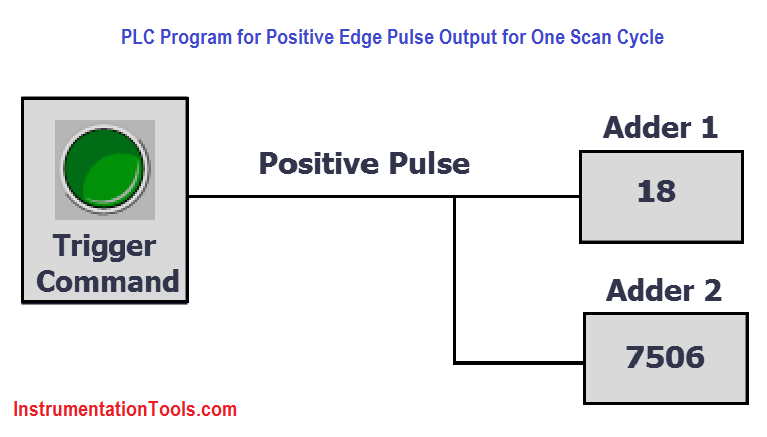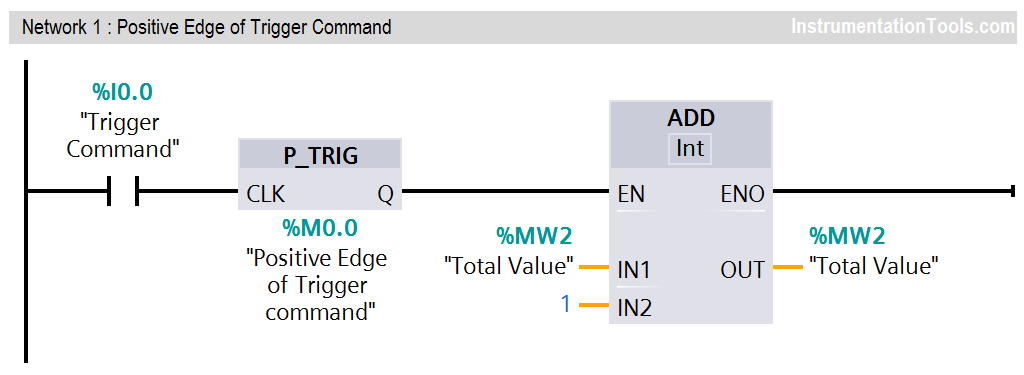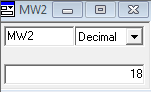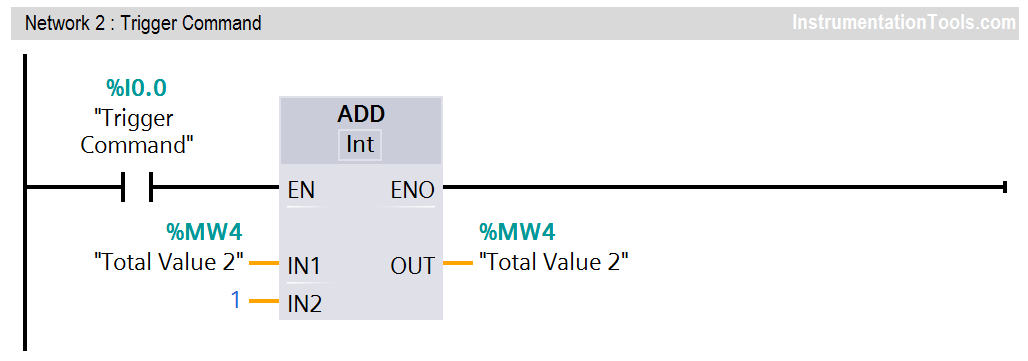Home » PLC Program for Positive Edge Pulse Output for One Scan Cycle

# PLC Program for Positive Edge Pulse Output for One Scan Cycle

## Positive Edge Pulse Output for One Scan Cycle

This is PLC Program for Positive edge pulse output for one scan cycle.

## Problem Description

In some applications, we need to run an operation/function based on external input signal. We can use a digital input as trigger command to activate that required function.

Sometimes we use positive transition of the digital input signal to trigger the command instead of continuous/full pulse digital input signal.

Here we consider an example of simple logic in which two registers values will be increment after receiving the trigger command. Each register have a preset value say value “1”. So on every trigger command, the adder register values will be increment by value “1”.

For adder 1 register we use positive edge (0 to 1) triggered input and for adder 2 register we use simple digital input  (0 to 1 & 1 to 0) signal. We see the advantages and disadvantages of using triggering command with and without using positive edge.

We can use the same logic in other applications like Zeroing the register values, forcing the register values with defined value with little logic modification etc.

### Problem Diagram### Problem Solution

• We can solve these types of problem by positive edge or rising edge of the digital input.
• Here we will consider S7-300 PLC for programming, so we can monitor the value and simulate it. We can use PLC SIM for simulation purpose.
• Here we have considered one simple example. In this example we will consider “Adder 1” register which will add value “1” when transition occurs from 0 to 1 of the trigger command. The register value will be incremented by value 1 after each triggering.
• For “Adder 2” register, the value will be incremented after receiving the digital input. Here, we are not used the positive edge triggering.

### List of Inputs/Outputs

#### Inputs List

• Trigger Command : I0.0

#### Memory Coil

• Positive Edge of trigger command : M0.0
• Total Value : MW2
• Total Value 2 : MW4

### PLC Ladder diagram for Positive edge pulse output for one scan cycle

#### Network 1 :

The initial value of “Adder 1″register is zero. After giving positive edge triggering command for 18 times, the output will be value 18 as it increments by value “1”.Simulation (PLCSIM-300) for trigger command with positive edge.#### Network 2 :

The initial value of “Adder 2″register is zero. After giving triggering command (without positive/negative edge) for 18 times, the output will come some random number (say 7506) instead of value 18 as trigger command directly received.Simulation (PLCSIM-300) for trigger command without positive edge.### Program Description

• In this application, we have used Siemens S7-300 PLC and TIA Portal Software for programming.
• Here we have considered two examples for positive edge explanation. Anyone can easily understand the concept.
• In Network 1, when trigger command (I0.0) is triggered then transition will occur from 0 to 1 and positive pulse instruction will be executed.
• Say “Adder 1″register will be stored with value “1” in MW0, if trigger command (I0.0) will be triggered then the value will be incremented by “1”.
• Here for example, we have triggered 18 times when adder 1 is zero, so adder added 18 in total Value (MW0)
• Another example we have taken in Network 2,  without using positive pulse. so here you can see the result.
• Say, We have pressed or triggered 18 times but it added 7506 (this is random value it can be different during simulation) in total Value 2 (MW4) so it is not proper addition. Because one pulse have rising or falling edges / positive or negative pulses (o to 1 and 1 to 0).
• Here also we have used PLC SIM for simulation, so we can simulate the total addition. In first network we have added positive edge so simulator is showing 18. In second network we have added trigger command without positive edge so it is showing some random value.
• This is the concept of positive edge, we can use this positive edge during any programming application.
• Above program and simulation is only for explanation purpose and simulation value can be different at simulation time.

### Runtime Test CasesNote : The above PLC Logic provided for basic idea about application of Positive edge trigger command in PLC Logic. The Logic is limited and not complete application.

#### How a PLC do the Scaling for a Sensor ?

This website uses cookies to improve your experience. We'll assume you're ok with this, but you can opt-out if you wish. Accept Read More

WordPress Image Lightbox

Send this to a friend